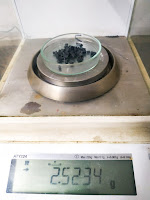# Determination of Density of Rubber & Rubber Accessories

## Pyknometer  ( Density bottle )

Apparatus
• Pycnometer (Density bottle)• Analytical Balance

### Reagent

• Distilled water

### Procedure

• Cut piece rubber sample
• Weight at least 2.5 gm• weight of density bottle, cut piece sample and water ( Use hot water )

• weight of Density bottle and water

Density of rubber (g/cm³) = M / M3 - M + M1
=  2.5234 / 44.7098 - 45.4444 + 2.5234
= 1.41 g/cm³
where
• M1 - Weight of sample
• M  Weight of Density bottle,sample and water
• M-  Weight of  density bottle and water

## 2. Dimension (mass/volume)

Apparatus
• Vernier Scale

Apparatus
• Analytical Balance

### Reagent

• Distilled water

• Tare Analytical balance (after setup see pic)

• weight of sample in air (gm)

Calculation

Density of rubber (g/cc) = M / M1  - M2
=  2.9291 / 2.9291 - 0.8608
= 1.41 g/cc
where
• M- Weight of sample in air
• M- Weight of sample in water

Reference - IS 3400 part- 9 - Method for Determination density  of Rubber materials